International
Tables for
Crystallography
Volume D
Physical properties of crystals
Edited by A. Authier

International Tables for Crystallography (2006). Vol. D, ch. 1.1, p. 7

## Section 1.1.3.1.1. Linear forms

A. Authiera*

aInstitut de Minéralogie et de la Physique des Milieux Condensés, Bâtiment 7, 140 rue de Lourmel, 75015 Paris, France
Correspondence e-mail: aauthier@wanadoo.fr

#### 1.1.3.1.1. Linear forms

| top | pdf |

A linear form in the spaceis written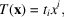where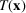is independent of the chosen basis and the's are the coordinates of T in the dual basis. Let us consider now a bilinear form in the product space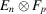of two vector spaces with n and p dimensions, respectively: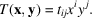The np quantities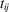's are, by definition, the components of a tensor of rank 2 and the form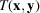is invariant if one changes the basis in the space. The tensoris said to be twice covariant. It is also possible to construct a bilinear form by replacing the spacesandby their respective conjugatesand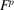. Thus, one writes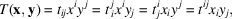where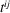is the doubly contravariant form of the tensor, whereas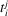andare mixed, once covariant and once contravariant.

We can generalize by defining in the same way tensors of rank 3 or higher by using trilinear or multilinear forms. A vector is a tensor of rank 1, and a scalar is a tensor of rank 0.